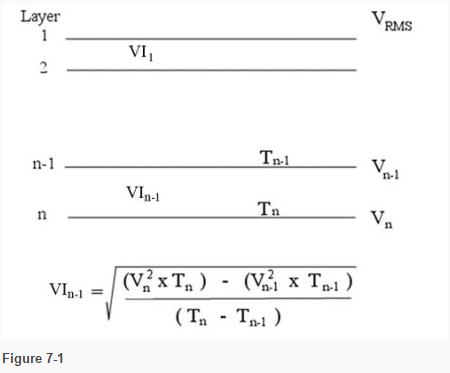6 Jan, 2016

# How Do We Use the Results from Velocity Analysis in our Seismic Data?Seismic velocities derived from moveout velocity analysis can be used to interpolate between wells or may be the only velocity information available in a new basin. This white paper will explore how we use the results from the velocity analyses, make corrections for lag, and account for floating datum.

Using the Results from the Velocity Analyses

Assuming the vertical velocities from the gathers are RMS velocities, these can be used for depth conversion with a little manipulation. If the effects of anisotropy have not been removed from the stacking velocities, they will need to be slowed down, maybe by 10-15%. If the reflectors are reasonably planar and horizontal, the RMS velocities can be converted to interval velocities using the DIX equation:Here, VIn-1 is the Interval RMS Velocity of the n-1th interval, which is not the same as the interval velocity from a well.

The RMS velocities can be turned into average velocities in two ways:

i. Reduce by 3-5% depending on the geological province

ii. Calculate VI (RMS) for all intervals between velocity-time pairs, derive

depths and calculate Va.

Correction for Lag

This is important when we need to calculate velocities for small intervals. The lag to be corrected is the difference between reflection onset, wavelet time-zero and picked time, or envelope maximum.

In velocity analysis, each time-velocity pair is generally picked on the highest amplitude part of a reflection; if there is any lag, this results in a calculated velocity which, although correct for stacking, is lower than the value corresponding to the observed moveout and the true reflection time.

The fractional error dV/V is approximately equal to either –dTo/2To or –dTo/To (where To = zero-offset reflection time), depending on whether the lag is constant across the gather or proportional to offset.

Moreover, this reduced velocity is assigned to a time value increased by the lag. Hence lag biases velocity functions towards low values (but not by a lot – about 1%).

The other effect is in the interpolation of digitized horizon times. If the digitized time is later than the true reflection time, interpolation will overestimate the velocity.

Example of the Effect of Wavelet Lag on Interval Velocity

Assume:

a) A minimum-phase wavelet with a lag of 20 milliseconds between onset and first trough

b) Reflections are picked in the major trough (peak if –ve reflection coefficient), the first stable loop

The base Miocene reflector is at 1.0s, stacking velocity Vs = 2200 m/s.

At two locations on the seismic, the picks are as follows:

 Location Base Miocene Base Miocene Interval T msec Vs m/s Vi m/s Loc A 970 2160 2475 lag corrected 950 2185 2520 (+2%) Loc B 1180 2200 2565 lag corrected 1160 2225 2615 (+2%)

So a lag of 20msec gives a velocity error in Vs of ~1%, requiring a correction of about +25 m/s to give the nmo velocity.

Floating Datum

Onshore, the seismic datum to which sections are corrected may be tens or hundreds of metres below (or above) ground level.

Moveout velocities, however, must be related to times measured from the ground level in order for the moveout equation to be applicable. Consequently, after the unstacked traces have received static corrections, each gather is re-adjusted back to a datum near the ground surface before velocity analysis and moveout correction.

This floating datum is usually an approximation to the actual ground surface. After stack, the traces are corrected to the seismic datum.

Floating datum is very important when stacking velocities are to be interpolated to horizon times; the times must be adjusted to the floating datum before velocity interpolation. Preferably, depths should be calculated relative to the floating datum as well, avoiding any doubt over what is actually being measured.

To view related PetroSkills courses, please see our Geophysics progression map.